This document is part of the HTML publication "An Introduction to the Imperative Part of C++"

The original version was produced by Rob Miller at Imperial College London, September 1996.

Version 1.1 (modified by David Clark at Imperial College London, September 1997)

Version 1.2 (modified by Bob White at Imperial College London, September 1998)

Version 1.3, 1.4, 2.0, ..., 2.21 (modified by William Knottenbelt at Imperial College London, September 1999-September 2022)

# 6. Arrays and Strings

## 6.1 The Basic Idea and Notation

Although we have already seen how to store large amounts of data in files, we have as yet no convenient way to manipulate such data from within programs. For example, we might want to write a program that inputs and then ranks or sorts a long list of numbers. C++ provides a structured data type called an array to facilitate this kind of task. The use of arrays permits us to set aside a group of memory locations (i.e. a group of variables) that we can then manipulate as a single entity, but that at the same time gives us direct access to any individual component. Arrays are simple examples of structured data types - they are effectively just lists of variables all of the same data type ("int", "char" or whatever). Later in the course you will learn how to construct more complicated compound data structures.

### Declaring an array

The general syntax for an array declaration is:

```	<component type>  <variable identifier>[<integer value>];
```

For example, suppose we are writing a program to manipulate data concerning the number of hours a group of 6 employees have worked in a particular week. We might start the program with the array declaration:

```	int hours;
```

or better,

```	const int NO_OF_EMPLOYEES = 6;
int hours[NO_OF_EMPLOYEES];
```

Indeed, if we are going to use a number of such arrays in our program, we can even use a type definition:

```	const int NO_OF_EMPLOYEES = 6;
typedef int Hours_array[NO_OF_EMPLOYEES];
Hours_array hours;
Hours_array hours_week_two;
```

In each case, we end up with 6 variables of type "int" with identifiers

```	hours   hours   hours   hours   hours   hours
```

Each of these is referred to as an element or component of the array. The numbers 0, ..., 5 are the indexes or subscripts of the components. An important feature of these 6 variables is that they are allocated consecutive memory locations in the computer. We can picture this as: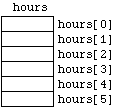Figure 6.1.1

### Assignment Statements and Expressions with Array Elements

Having declared our array, we can treat the individual elements just like ordinary variables (of type "int" in the particular example above). In particular, we can write assignment statements such as

```	hours = 34;
hours = hours/2;
```

and use them in logical expressions, e.g.

```	if (number < 4 && hours[number] >= 40) { ...
```

A common way to assign values to an array is using a "for" or "while" loop. The following program prompts the user for the number of hours that each employee has worked. It is more natural to number employees from 1 to 6 than from 0 to 5, but it is important to remember that array indexes always start from 0. Hence the program subtracts 1 from each employee number to obtain the corresponding array index.

```	#include <iostream>
using namespace std;

const int NO_OF_EMPLOYEES = 6;
typedef int Hours_array[NO_OF_EMPLOYEES];

int main()
{
Hours_array hours;
int count;

for (count = 1 ; count <= NO_OF_EMPLOYEES ; count++)
{
cout << "Enter hours for employee number " << count << ": ";
cin >> hours[count - 1];
}

return 0;
}
```

A typical run might produce the following input/output:

```	Enter hours for employee number 1: 38
Enter hours for employee number 2: 42
Enter hours for employee number 3: 29
Enter hours for employee number 4: 35
Enter hours for employee number 5: 38
Enter hours for employee number 6: 37
```

in which case our block of variables would then be in the state: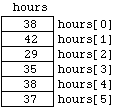Figure 6.1.2

It is instructive to consider what would have happened had we forgotten to subtract 1 from the variable "count" in the "cin ..." statement (within the "for" loop) in Program 6.1.1. Unlike some languages, C++ does not do range bound error checking, so we would have simply ended up in the state: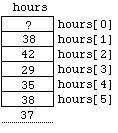Figure 6.1.3 - A Range Bound Error

In other words, C++ would have simply put the value "37" into the next integer-sized chunk of memory located after the memory block set aside for the array "hours". This is a very undesirable situation - the compiler might have already reserved this chunk of memory for another variable (perhaps, for example, for the variable "count").

Array elements can be of data types other than "int". Here's a program that prints itself out backwards on the screen, using an array of type "char".

```	#include <iostream>
#include <fstream>

using namespace std;

const int MAX = 1000;
typedef char File_array[MAX];

int main()
{
char character;
File_array file;
int count;
ifstream in_stream;

in_stream.open("6-1-2.cpp");
in_stream.get(character);
for (count = 0 ; ! in_stream.eof() && count < MAX ; count++)
{
file[count] = character;
in_stream.get(character);
}
in_stream.close();

while (count > 0)
cout << file[--count];

return 0;
}
```

Program 6.1.2

Note the use of the condition "... && count < MAX ; ..." in the head of the "for" loop, to avoid the possibility of a range bound error.

## 6.2 Arrays as Parameters in Functions

Functions can be used with array parameters to maintain a structured design. Here is a definition of an example function which returns the average hours worked, given an array of type "Hours_array" from Program 6.1.1

```	float average(Hours_array hrs)
{
float total = 0;
int count;
for (count = 0 ; count < NO_OF_EMPLOYEES ; count++)
total += float(hrs[count]);
return (total / NO_OF_EMPLOYEES);
}
```

Fragment of Program 6.2.1

We could make this function more general by including a second parameter for the length of the array:

```	float average(int list[], int length)
{
float total = 0;
int count;
for (count = 0 ; count < length ; count++)
total += float(list[count]);
return (total / length);
}
```

It is quite common to pass the array length to a function along with an array parameter, since the syntax for an array parameter (such as "int list[]" above) doesn't include the array's length.

Although array parameters are not declared with an "&" character in function declarations and definitions, they are effectively reference parameters (rather than value parameters). In other words, when they execute, functions do not make private copies of the arrays they are passed (this would potentially be very expensive in terms of memory). Hence, like the reference parameters we have seen in Lecture 3, arrays can be permanently changed when passed as arguments to functions. For example, after a call to the following function, each element in the third array argument is equal to the sum of the corresponding two elements in the first and second arguments:

```	void add_lists(int first[], int second[], int total[], int length)
{
int count;
for (count = 0 ; count < length ; count++)
total[count] = first[count] + second[count];
}
```

Fragment of Program 6.2.2

As a safety measure, we can add the modifier "const" in the function head:

```	void add_lists(const int fst[], const int snd[], int tot[], int len)
{
int count;
for (count = 0 ; count < len; count++)
tot[count] = fst[count] + snd[count];
}
```

The compiler will then not accept any statements within the function's definition which potentially modify the elements of the arrays "fst" or "snd". Indeed, the restriction imposed by the "const" modifier when used in this context is stronger than really needed in some situations. For example, the following two function definitions will not be accepted by the compiler:

```	void no_effect(const int list[])
{
do_nothing(list);
}

void do_nothing(int list[])
{
;
}
```

Fragment of Program 6.2.3

This is because, although we can see that "do_nothing(...)" does nothing, its head doesn't include the modifier "const", and the compiler only looks at the head of "do_nothing(...)" when checking to see if the call to this function from within "no_effect(...)" is legal.

## 6.3 Sorting Arrays

Arrays often need to be sorted in either ascending or descending order. There are many well known methods for doing this; the quick sort algorithm is among the most efficient. This section briefly describes one of the easiest sorting methods called the selection sort.

The basic idea of selection sort is:

For each index position I in turn:

1. Find the smallest data value in the array from positions I to (Length - 1), where "Length" is the number of data values stored.
2. Exchange the smallest value with the value at position I.

To see how selection works, consider an array of five integer values, declared as

```	int a;
```

and initially in the state: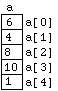Figure 6.3.1

Selection sort takes the array through the following sequence of states: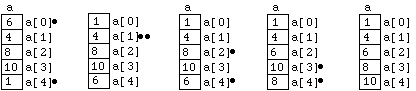Figure 6.3.2

Each state is generated from the previous one by swapping the two elements of the array marked with a "bullet".

We can code this procedure in C++ with three functions. The top level function "selection_sort(...)" (which takes and array and an integer argument) sorts its first (array) argument by first calling the function "minimum_from(array,position,length)", which returns the index of the smallest element in "array" which is positioned at or after the index "position". It then swaps values according to the specification above, using the "swap(...)" function:

```	void selection_sort(int a[], int length)
{
for (int count = 0 ; count < length - 1 ; count++)
swap(a[count],a[minimum_from(a,count,length)]);
}

int minimum_from(int a[], int position, int length)
{
int min_index = position;

for (int count = position + 1 ; count < length ; count ++)
if (a[count] < a[min_index])
min_index = count;

return min_index;
}

void swap(int& first, int& second)
{
int temp = first;
first = second;
second = temp;
}
```

Fragment of Program 6.3.1

## 6.4 Two-dimensional Arrays

Arrays can have more than one dimension. In this section we briefly examine the use of two-dimensional arrays to represent two-dimensional structures such as screen bitmaps or nxm matrices of integers.

A bitmap consists of a grid of Boolean values representing the state of the dots or pixels on a screen. "True" means "on" or that the pixel is white; "False" means "off" or the pixel is black. Let's suppose the screen is 639 pixels wide and 449 pixels high. We can declare the corresponding array as follows:

```	enum Logical {False, True};
const int SCREEN_HEIGHT = 449;
const int SCREEN_WIDTH = 639;
Logical screen[SCREEN_HEIGHT][SCREEN_WIDTH];
```

References to individual data elements within the array "screen" simply use two index values. For example, the following statement assigns the value "True" to the cell (pixel) in row 4, column 2 of the array.

```	screen = True;
```

All of the discussion in Section 6.2 about one-dimensional arrays as parameters in functions also applies to two-dimensional arrays, but with one additional peculiarity. In function declarations and in the heads of function definitions, the size of the first dimension of a multidimensional array parameter is not given (inside the "[]" brackets), but the sizes of all the other dimensions are given. Hence, for example, the following is a correct form for a function which sets all the screen pixels to black:

```	void clear_bitmap(Logical bitmap[][SCREEN_WIDTH], int screen_height)
{
for (int row = 0 ; row < screen_height ; row++)
for (int column = 0 ; column < SCREEN_WIDTH; column++)
bitmap[row][column] = False;
}
```

## 6.5 Strings

We have already been using string values, such as ""Enter age: "", in programs involving output to the screen. In C++ you can store and manipulate such values in string variables, which are really just arrays of characters, but used in a particular way.

### The Sentinel String Character '\0'

The key point is that, to use the special functions associated with strings, string values can only be stored in string variables whose length is at least 1 greater than the length (in characters) of the value. This is because extra space must be left at the end to store the sentinel string character "'\0'" which marks the end of the string value. For example, the following two arrays both contain all the characters in the string value ""Enter age: "", but only the array on the left contains a proper string representation.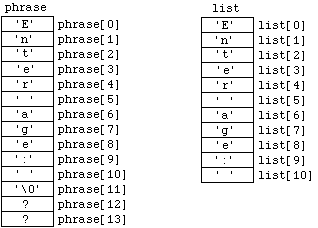Figure 6.5.1

In other words, although both "phrase" and "list" are arrays of characters, only "phrase" is big enough to contain the string value ""Enter age: "". We don't care what characters are stored in the variables "phrase" and "phrase", because all the string functions introduced below ignore characters after the "'\0'".

### String Variable Declarations and Assignments

String variables can be declared just like other arrays:

```	char phrase;
```

String arrays can be initialised or partially initialised at the same time as being declared, using a list of values enclosed in "{}" braces (the same is true of arrays of other data types). For example, the statement

```	char phrase = {'E','n','t','e','r',' ','a','g','e',':',' ','\0'};
```

both declares the array "phrase" and initialises it to the state in Figure 6.5.1. The statement

```	char phrase = "Enter age: ";
```

is equivalent. If the "14" is omitted, an array will be created just large enough to contain both the value ""Enter age: "" and the sentinel character "'\0'", so that the two statements

```	char phrase[] = {'E','n','t','e','r',' ','a','g','e',':',' ','\0'};
char phrase[] = "Enter age: ";
```

are equivalent both to each other and to the statement

```	char phrase = "Enter age: ";
```

However, it is important to remember that string variables are arrays, so we cannot just make assignments and comparisons using the operators "=" and "==". We cannot, for example, simply write

```	phrase = "You typed: ";
```

Instead, we can use a special set of functions for string assignment and comparison.

### Some Predefined String Functions

The library cstring (old style header string.h) contains a number of useful functions for string operations. We will assume that the program fragments discussed below are embedded in programs containing the "include" statement

```	#include<cstring>
```

Given the string variable "a_string", we can copy a specific string value or the contents of another string to it using the two argument function "strcpy(...)". Hence the statement

```	strcpy(a_string, "You typed: ");
```

assigns the first 11 elements of "a_string" to the respective characters in ""You typed: "", and assigns the sentinel character "'\0'" to the 12th element. The call

```	strcpy(a_string, another_string);
```

copies the string value stored in "another_string" to "a_string". But care has to be taken with this function. If "a_string" is less than (1 + L), where L is the length of the string value currently stored in "another_string", the call to the function will cause a range bound error which will not be detected by the compiler.

We can, however, check the length of the value stored in "another_string" using the function "strlen(...)". The call "strlen(another_string)" returns the length of the current string stored in "another_string" (the character "'\0'" is not counted).

The comparison function "strcmp(...)" returns "False" (i.e. 0) if its two string arguments are the same, and the two argument function "strcat(...)" concatenates its second argument onto the end of its first argument. Program 6.5.1 illustrates the use of these functions. Again, care must be taken with "strcat(...)". C++ does not check that the first variable argument is big enough to contain the two concatenated strings, so that once again there is a danger of undetected range bound errors.

### String Input using "getline(...)"

Although the operator ">>" can be used to input strings (e.g. from the keyboard), its use is limited because of the way it deals with space characters. Supposing a program which includes the statements

```	...
...
cout << "Enter name: ";
cin >> a_string;
...
...
```

results in the input/output session

```	...
...
Enter name: Rob Miller
...
...
```

The string variable will then contain the string value ""Rob"", because the operator ">>" assumes that the space character signals the end of input. It is therefore often better to use the two argument function "getline(...)". For example, the statement

```	cin.getline(a_string,80);
```

allows the user to type in a string of up to 79 characters long, including spaces. (The extra element is for the sentinel character.) The following program illustrates the use of "getline(...)", "strcmp(...)", "strcpy(...)" and "strcat(...)":

```	#include <iostream>
#include <cstring>

using namespace std;

const int MAXIMUM_LENGTH = 80;

int main()
{
char first_string[MAXIMUM_LENGTH];
char second_string[MAXIMUM_LENGTH];

cout << "Enter first string: ";
cin.getline(first_string,MAXIMUM_LENGTH);
cout << "Enter second string: ";
cin.getline(second_string,MAXIMUM_LENGTH);

cout << "Before copying the strings were ";
if (strcmp(first_string,second_string))
cout << "not ";
cout << "the same.\n";

strcpy(first_string,second_string);

cout << "After copying the strings were ";
if (strcmp(first_string,second_string))
cout << "not ";
cout << "the same.\n";

strcat(first_string,second_string);

cout << "After concatenating, the first string is: ";
cout << first_string;

return 0;
}
```

Program 6.5.1

A example input/output session is:

```	Enter first string: Hello class.
Enter second string: Hello Rob.
Before copying the strings were not the same.
After copying the strings were the same.
After concatenating, the first string is: Hello Rob.Hello Rob.
```

## 6.6 Summary

In this lecture we have seen how a list of variables of the same data type may be represented as an array. We have seen how arrays may be passed as parameters to functions, and we have seen a simple algorithm for sorting arrays. We have also briefly discussed the notion of a two-dimensional array. Finally, we have seen that C++ provides some special functions to manipulate strings, which are simply arrays of characters always including a special sentinel character. The material here is also covered in more detail in Savitch, Chapter 7 and Chapter 8.